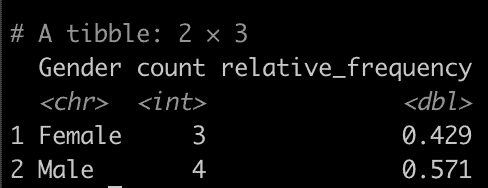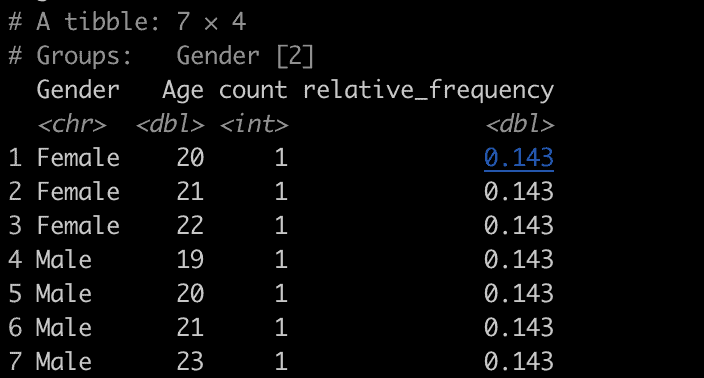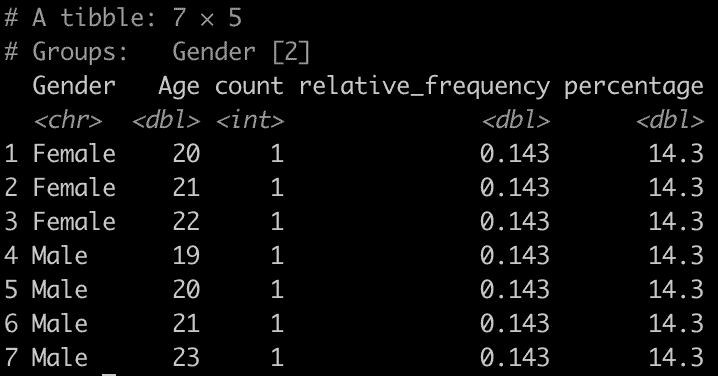# How to Calculate Relative Frequencies Using dplyr in R

To calculate relative frequencies using the dplyr package in R, you typically follow these steps:

1. Count the number of occurrences of each value or group.
2. Calculate the sum of all counts to get the total.
3. Divide each count by the total to get the relative frequency.

## Example 1: Calculating the Relative Frequency of One Variable

``````library(dplyr)

df <- data.frame(
Age = c(20, 21, 19, 22, 23, 20, 21),
Gender = c("Male", "Female", "Male", "Female", "Male", "Female", "Male"),
Score = c(85, 90, 88, 78, 92, 80, 87)
)

df %>%
group_by(Gender) %>%
summarise(count = n()) %>%
mutate(relative_frequency = count / sum(count))``````

OutputIn this code:

1. group_by(Gender) groups the data by Gender.
2. summarise(count = n()) counts the number of rows in each gender group.
3. mutate(relative_frequency = count/sum (count)) calculates the relative frequency by dividing each count by the total count.

## Example 2: Calculating the Relative Frequency of Multiple Variables

To calculate the relative frequency of multiple variables simultaneously using dplyr, you can use the group_by() function with multiple grouping variables followed by the summarise() and mutate() functions.

``````library(dplyr)

df <- data.frame(
Age = c(20, 21, 19, 22, 23, 20, 21),
Gender = c("Male", "Female", "Male", "Female", "Male", "Female", "Male"),
Score = c(85, 90, 88, 78, 92, 80, 87)
)

df %>%
group_by(Gender, Age) %>%
summarise(count = n()) %>%
mutate(relative_frequency = count / nrow(df))``````

OutputIn this code:

1. group_by(Gender, Age) groups the data by Gender and Age.
2. summarise(count = n()) counts the number of rows for each combination of Gender and Age.
3. mutate(relative_frequency = count / nrow(df)) calculates the relative frequency for each combination by dividing each count by the total number of rows in the dataframe.

The resulting dataframe will contain each unique combination of `Gender` and `Age` along with its corresponding relative frequency.

## Example 3: Displaying Relative Frequencies as Percentages

To display relative frequencies as percentages, you can multiply the relative frequencies by 100. Continuing from the previous example, where we calculated the relative frequencies for both Gender and Age in the dataframe, you can add mutate() function to convert the relative frequencies into percentages:

``````library(dplyr)

df <- data.frame(
Age = c(20, 21, 19, 22, 23, 20, 21),
Gender = c("Male", "Female", "Male", "Female", "Male", "Female", "Male"),
Score = c(85, 90, 88, 78, 92, 80, 87)
)

df %>%
group_by(Gender, Age) %>%
summarise(count = n()) %>%
mutate(
relative_frequency = count / nrow(df),
percentage = relative_frequency * 100
)``````

OutputThat’s it!

## Related posts

Find the Maximum Value By Group in R

Summary Statistics By Group in R

Weighted Mean in R

Conditional Mean in R

Unique values by a group in R

Sum by Group in R